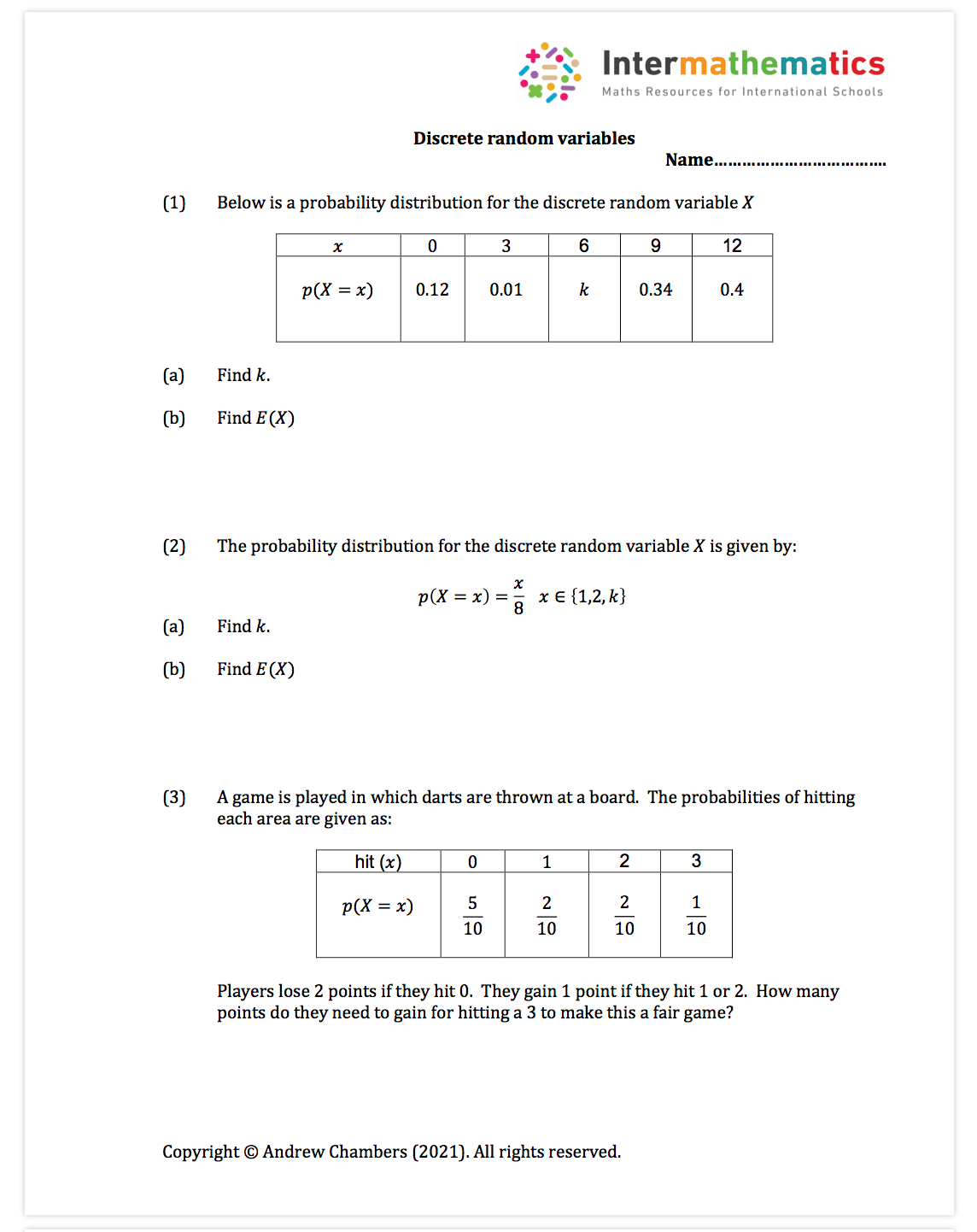# Statistics and Probability Free content

Statistics and Probability content: This content is designed to help with the whole of the statistics and probability sections of the syllabus.

Worksheets in premium set: 47 worksheets (around 100 pdf pages of questions and around 150 pdf pages of fully typed solutions).

I have used Geogebra and Desmos throughout to help illustrate the statistics and data.

Example probability worksheet: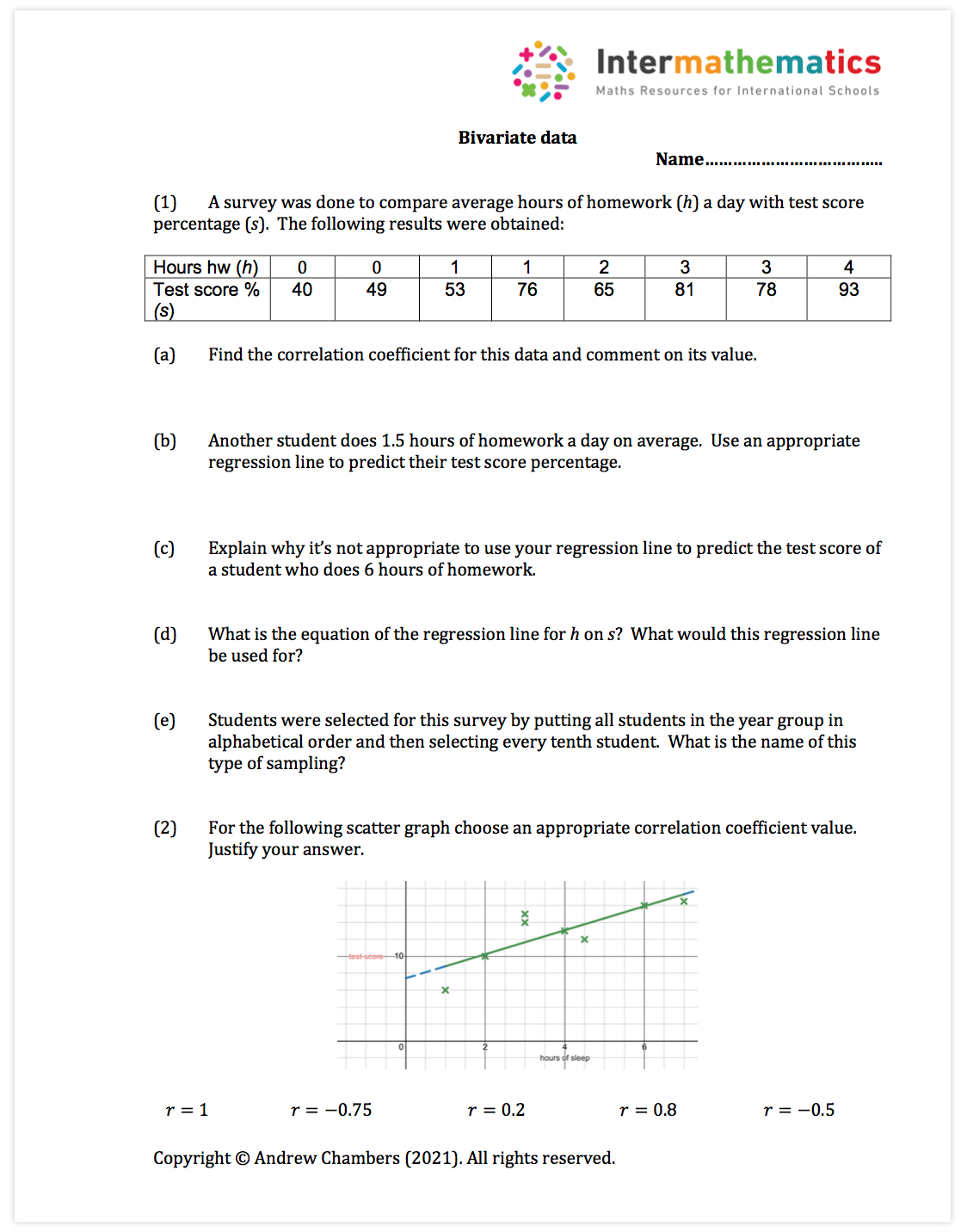Student activity example (treasure hunt):Student investigation example: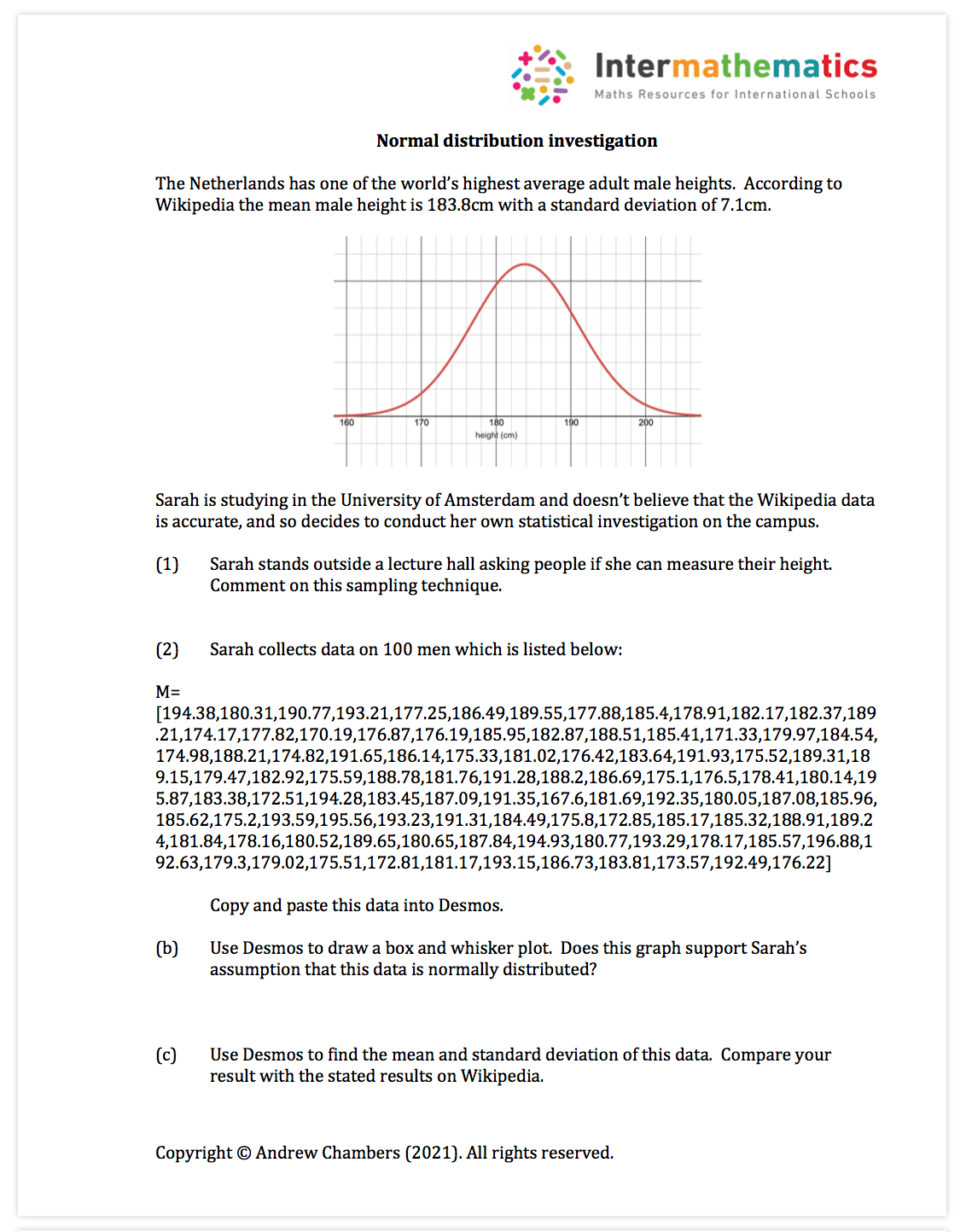Paper 3 investigation example: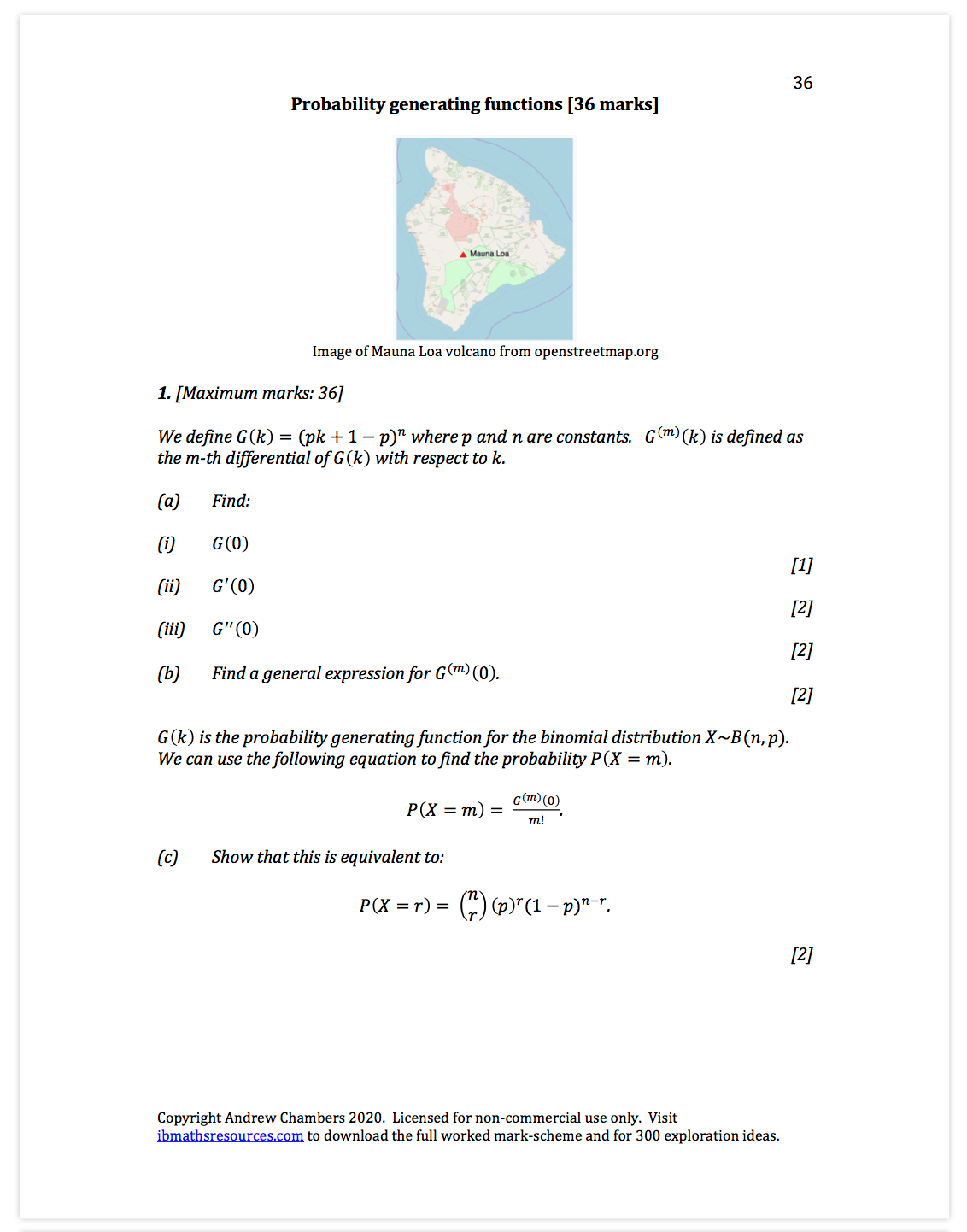Student activity example (treasure hunt):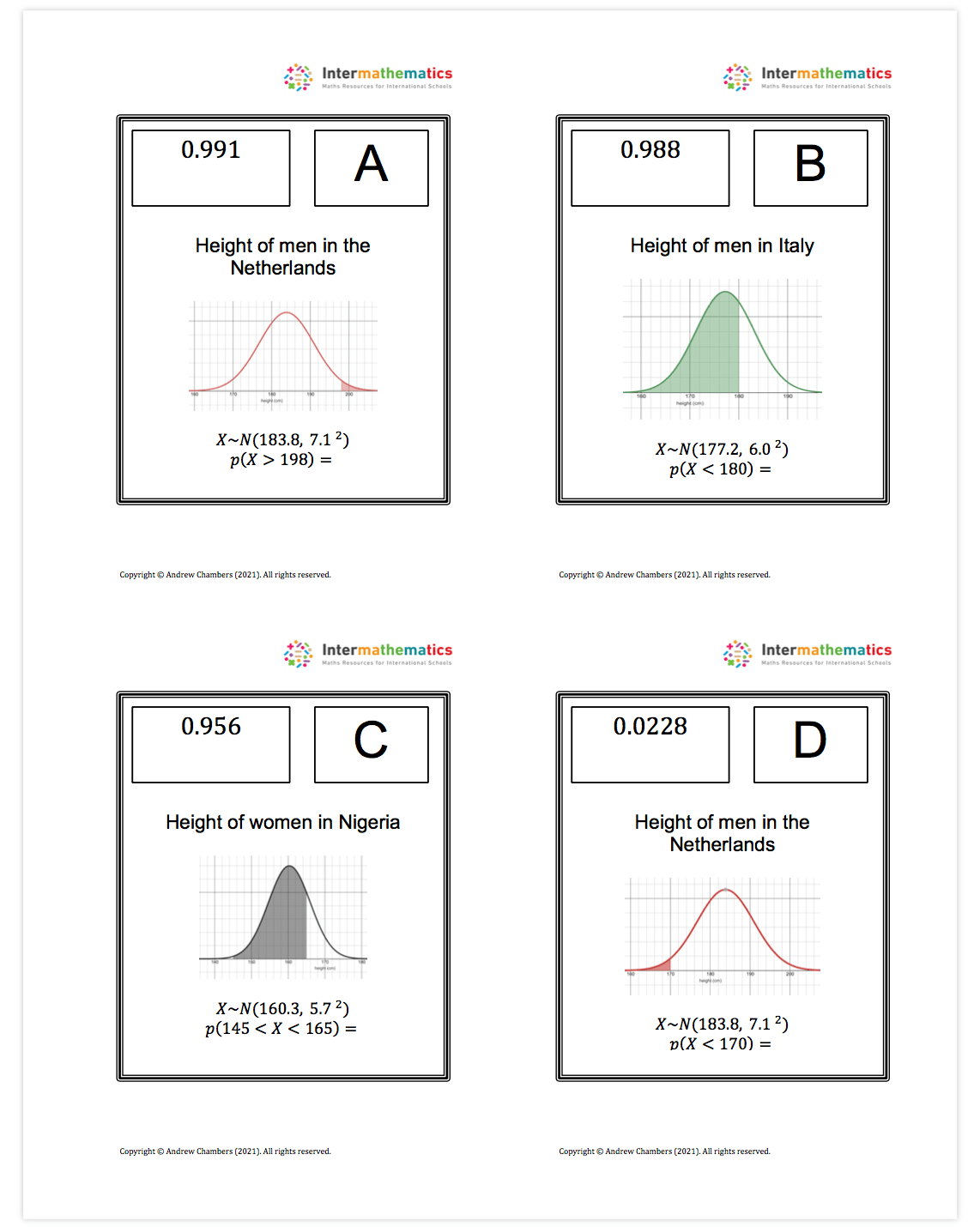Worksheet example: